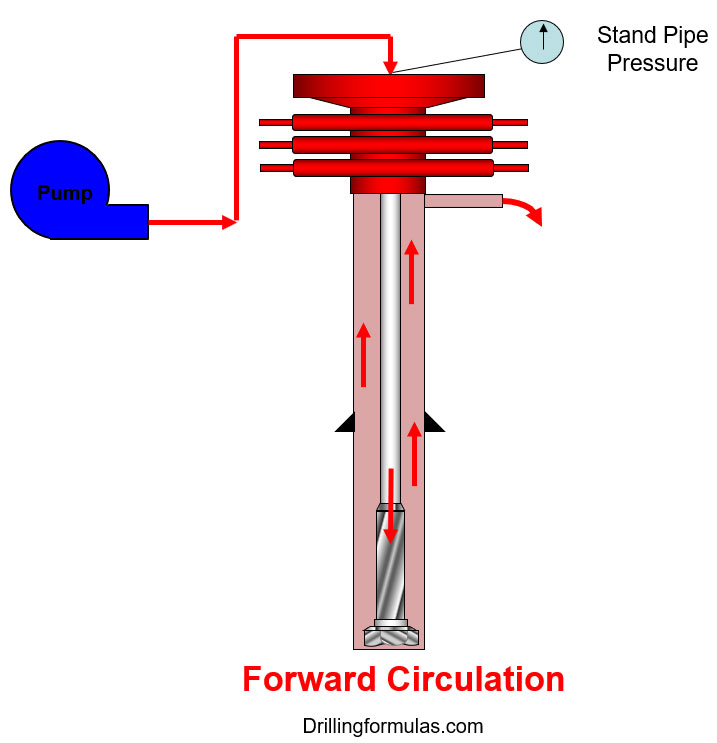# Effect of Frictional Pressure on ECD while Forward Circulation

In this article, we will describe the effect of friction pressure on bottom hole pressure and equivalent circulating density while performing forward circulation.

### What is forward circulation?

It is the typical circulating path which is from a mud pump into drill pipe. Mud is pumped down into drill string / BHA and come out of a bit.  Then, the mud is flown up the annulus and return back to surface as you can see in the diagram below (Figure 1).Figure 1 – Forward Circulation Path

## Under a static condition:

The bottom hole pressure is equal to hydrostatic pressure from the drilling fluid.

Bottom Hole Pressure (BHP)  = Hydrostatic Pressure (HP)

## Under a dynamic condition:

Stand pipe pressure equates to summation of pressure loss of whole system.Figure 2 – Pressure loss component during forward circulation

SPP = FrPds + FrPbha + FrPbit + FrPann

Where;

SPP = Stand Pipe Pressure

FrPds = Pressure loss in drill string

FrPbha = Pressure loss in BHA

FrPbit= Pressure loss across the bit

FrPann = Pressure loss in Annulus

The friction pressure acts opposite way while fluid is being moved; therefore, if you look at the annulus side, you will be able to determine the bottom hole pressure at the dynamic condition as per the equation below.

Bottom Hole Pressure (BHP) = Hydrostatic Pressure (HP) + Pressure Loss in Annulus (FrPann)

Under the dynamic condition, the only effect on the bottom hole pressure is the pressure loss in the annulus. This is the reason why the ECD while forward circulation is expressed like this;Where;

• ECD = Equivalent Circulating Density, ppg
• MW = Mud Weight in the well, ppg
• FrPann = Pressure loss in Annulus, psi
• TVD = True Vertical Depth, ft

References

Cormack, D. (2007). An introduction to well control calculations for drilling operations. 1st ed. Texas: Springer.

Crumpton, H. (2010). Well Control for Completions and Interventions. 1st ed. Texas: Gulf Publishing.

Grace, R. (2003). Blowout and well control handbook [recurso electrónico]. 1st ed. Paises Bajos: Gulf Professional Pub.

Grace, R. and Cudd, B. (1994). Advanced blowout & well control. 1st ed. Houston: Gulf Publishing Company.

Watson, D., Brittenham, T. and Moore, P. (2003). Advanced well control. 1st ed. Richardson, Tex.: Society of Petroleum Engineers.

Share the joyWorking in the oil field and loving to share knowledge.

### 14 Responses to Effect of Frictional Pressure on ECD while Forward Circulation

1.Ahmed says:

I have a question, why the pressure loss across the bit does not affect the bottomhole pressure?
If I reference the BHP to tubing site, shouldn’t be like this
BHP=pump pressure+hydrostatic pressure-pressure loss across the bit- pressure loss in the drillstring+pressure loss in BHA?

•DrillingFormulas.Com says:

It depends on which side you refer to. For example if you refer to drill pipe side, your equation is
BHP=pump pressure+hydrostatic pressure-pressure loss across the bit- pressure loss in the drillstring-pressure loss in BHA
or if you are reference to annulus side, your equitation will be
BHP = hydrostatic pressure + annular pressure loss.
Both of them are equal in terms of mathematics.

2.Ahmed says:

sorry i meant, minus pressure loss in BHA

3.Babak says:

Why does the annular pressure increase during the influx when gas moves upwards due to pressure release while the height of mud decreases?

•DrillingFormulas.Com says:

The annular pressure increase due to loss of hydrostatic pressure.
BHP = Hydrostatic Pressure + Surface Pressure. Once the gas is moving up, the gas volume expand therefore the well will lose the hydrostatic pressure. In order to balance formation pressure as stated in the equation, the surface pressure will increase.

4.zayed says:

In UBD Operation
BHP in annuals side will be :
Pbh = Mud hydrostatic + Annular fractional pressure + Choke pressure
is that correct?
Thank you

•DrillingFormulas.Com says:

Yes. This is correct.

5.raed says:

tnx for share us this information

6.Iakn says:

Considering the hole size and OD of the pipe is same throughtout the hole, would ECD in annulus be the same at TD and at say, in the middle?

•DrillingFormulas.Com says:

It is the same ECD.

7.James Hinton says:

Your ECD equation does not account for any fluid rheological effects. Does fluid rheology not affect the pressure loss within the annulus (and well)?

•DrillingFormulas.Com says:

James,

Yes. Rheology is one of contributing factors to the ECD. In this article, rheology is hinden the annulas pressure loss. We don’t have the details calculation in this article. You can find the detailed calculations in several posts in our website.

Best Regards,
Shyne

8.AKHI says:

Hi,
If we increase the mud weight, SPP will increase or decrease.
It shoud be increase right.
But some one told me it will decrease. But he doesn’t explain why.
•DrillingFormulas.Com says: